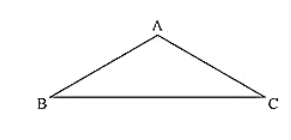# In a ΔABC, if ∠A = 120° and AB = AC. Find ∠B and ∠C.Question:

In a ΔABC, if ∠A = 120° and AB = AC. Find ∠B and ∠C.

Solution:

Consider a ΔABC

Given Mat ∠A = 120° and AB = AC and given to find ∠B and ∠C.

We can observe that ΔABC is an isosceles triangle since AB = AC

∠B = ∠C (i)

[Angles opposite to equal sides are equal]

We know that sum of angles in a triangle is equal to 180°

⟹ ∠A + ∠B + ∠C = 180°                                    [From (i)]

⟹ ∠A + ∠B + ∠B = 180°

⟹ 120° + 2∠B = 180°

⟹ 2∠B = 180° - 120°

⟹ ∠B = ∠C = 30°